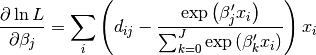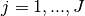# statsmodels.discrete.discrete_model.MNLogit.score¶

MNLogit.score(params)[source]

Score matrix for multinomial logit model log-likelihood

Parameters: params : array The parameters of the multinomial logit model. score : ndarray, (K * (J-1),) The 2-d score vector, i.e. the first derivative of the loglikelihood function, of the multinomial logit model evaluated at params.

NotesforIn the multinomial model the score matrix is K x J-1 but is returned as a flattened array to work with the solvers.